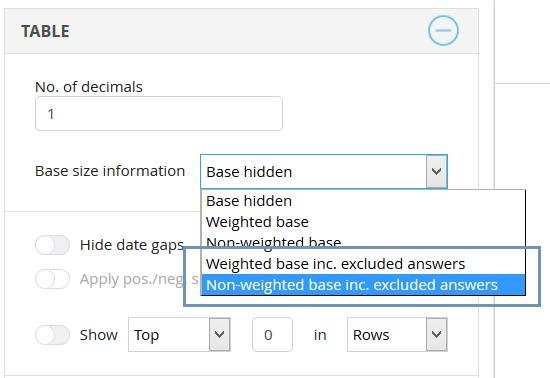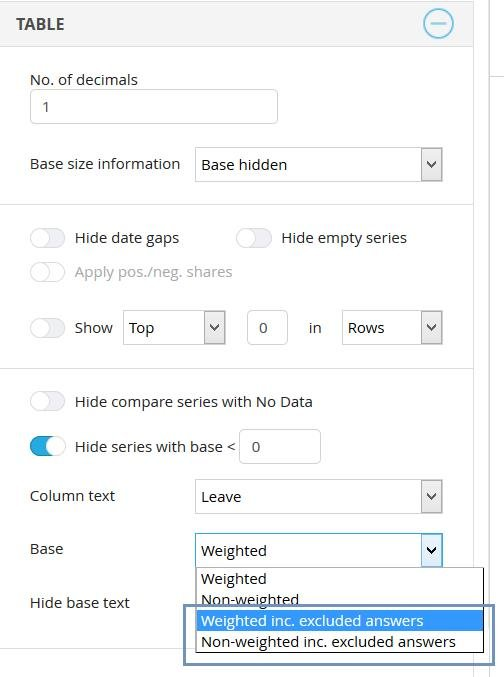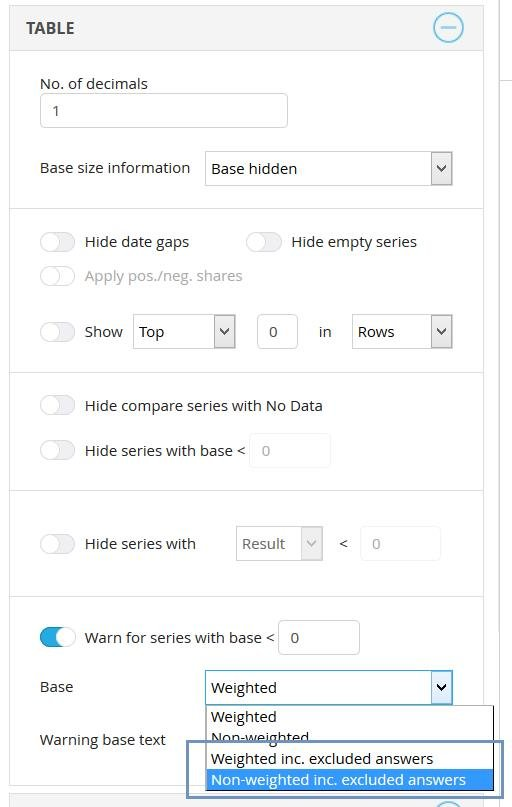When calculating a mean value result of a categorical question, an answer like “Don’t know” is usually excluded from the calculation (the settings which allow the exclusion of an answer from the mean value calculation is made in the Answer block page). When excluding an answer from the calculation, these respondents are not included in the base size displayed in the table or when determining if a result should be hidden or not due to a low base size.

There is an option available by which you can show a base size that also includes the respondents answering an excluded answer option, like “Don’t know.” This option can be applied to:

• The base size shown in the tables
• The base size used for hiding a result due to low base
• Warning due to low base

*The calculation of a result is not affected. The setting only affects the displayed base size and the base size used for hiding/warning due to low base size. The actual mean values calculated prior to introducing this option are not changed.

The base size options are shown in the regular selection lists where you can define if a base size should be shown or not. Since the new option can only be applied to mean value calculations of categorical questions, the options are only shown when this calculation type is selected. Also, the options are only shown if any answers in the project have been excluded from the mean value calculation. The image below shows the new options for the displayed base size.The image below shows the options for hiding results due to low base size.The image below shows the options for low base size warning.When option “Hide series with Result” is used it uses the calculation that has been selected for that table.
-if the table shows COUNT calculation, the option “Result” means Count
-if the table shows % calculation, the option “Result” means %
-if the table shows MEAN calculation, the option “Result” means MEAN